# Directly and inversely proportional- equationsPage 1

#### WATCH ALL SLIDES

Slide 1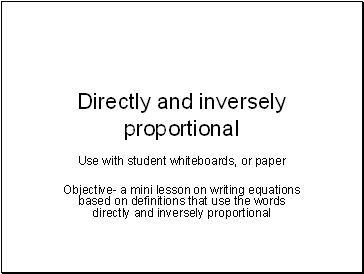## Directly and inversely proportional

Use with student whiteboards, or paper

Objective- a mini lesson on writing equations based on definitions that use the words directly and inversely proportional

Slide 2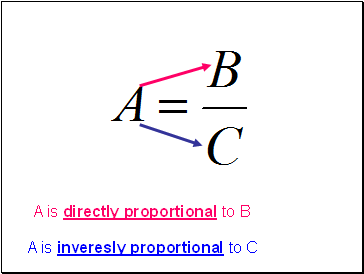A is directly proportional to B

A is inveresly proportional to C

Slide 3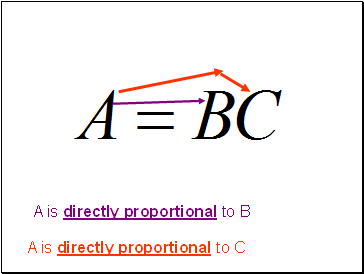A is directly proportional to B

A is directly proportional to C

Slide 4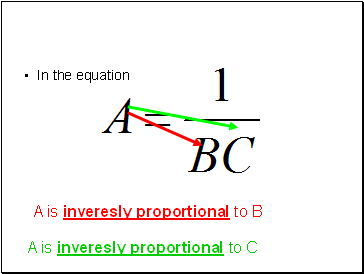In the equation

A is inveresly proportional to B

A is inveresly proportional to C

Slide 5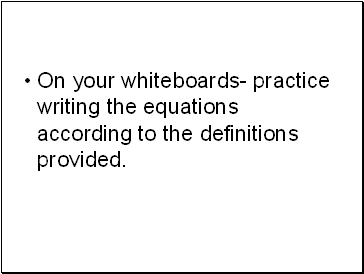On your whiteboards- practice writing the equations according to the definitions provided.

Slide 6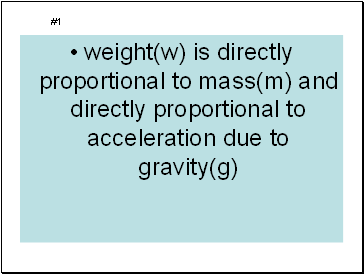weight(w) is directly proportional to mass(m) and directly proportional to acceleration due to gravity(g)

#1

Slide 7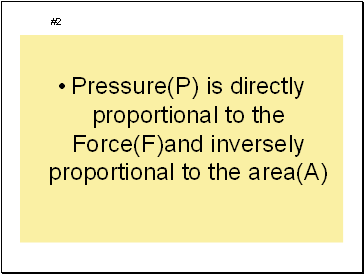Pressure(P) is directly proportional to the Force(F)and inversely proportional to the area(A)

#2

Slide 8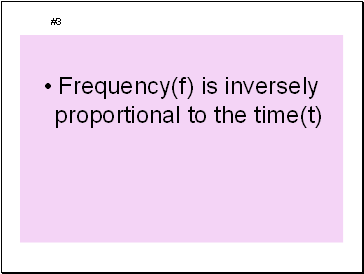Frequency(f) is inversely proportional to the time(t)

#3

Slide 9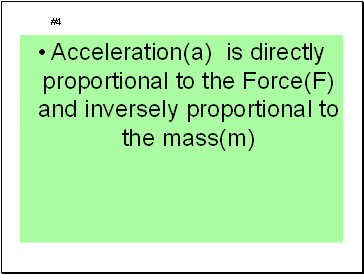Acceleration(a) is directly proportional to the Force(F) and inversely proportional to the mass(m)

#4

Slide 10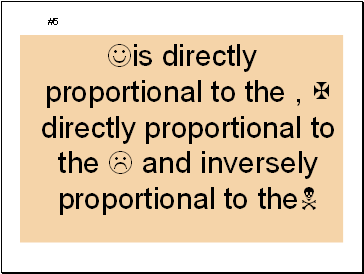Jis directly proportional to the , X directly proportional to the L and inversely proportional to theN

#5

Slide 11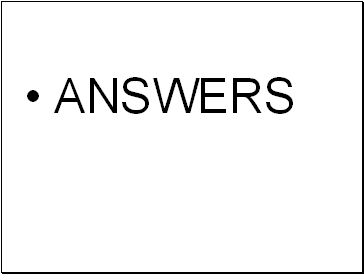ANSWERS

Slide 12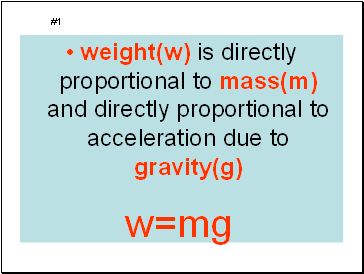weight(w) is directly proportional to mass(m) and directly proportional to acceleration due to gravity(g)

#1

w=mg

Slide 13Pressure(P) is directly proportional to the Force(F)and inversely proportional to the area(A)

#2

P=F/A

Slide 14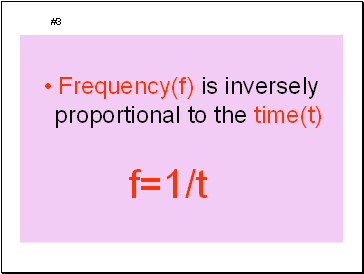Frequency(f) is inversely proportional to the time(t)

#3

f=1/t

Slide 15Acceleration(a) is directly proportional to the Force(F) and inversely proportional to the mass(m)

Go to page:
1  2

## Last added presentations

© 2010-2021 powerpoint presentations